Publicité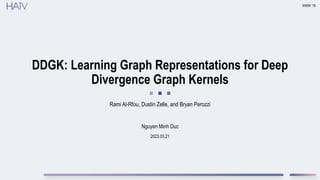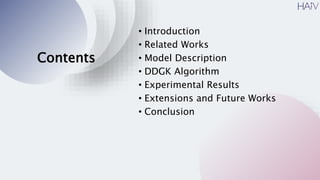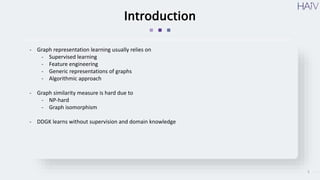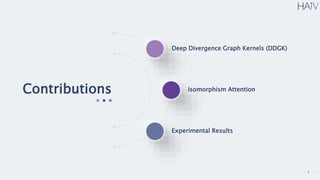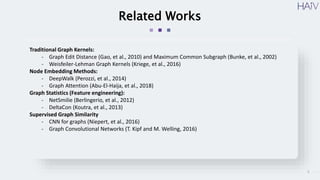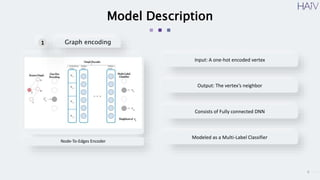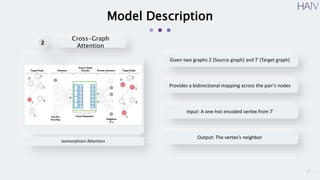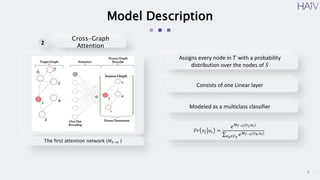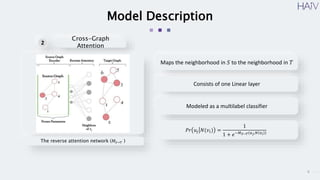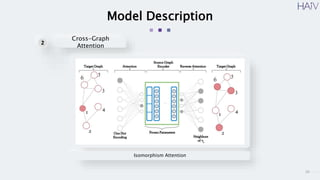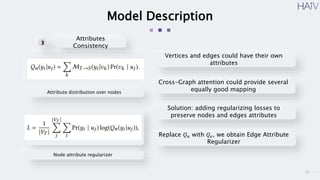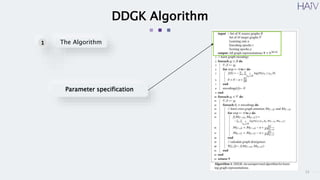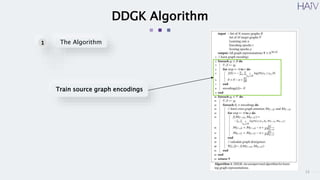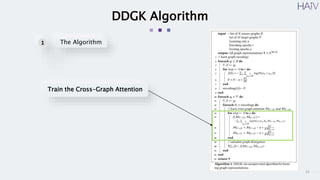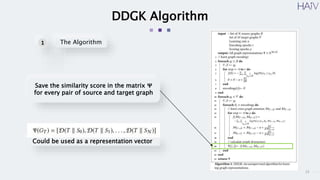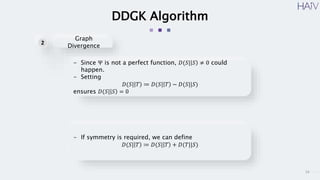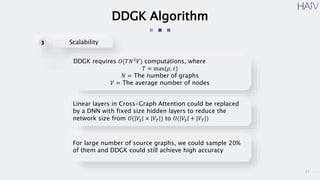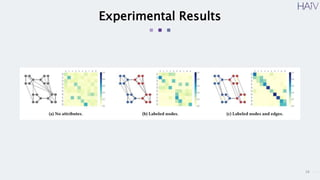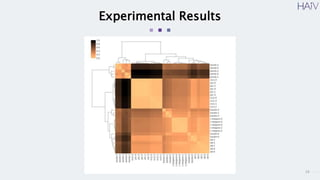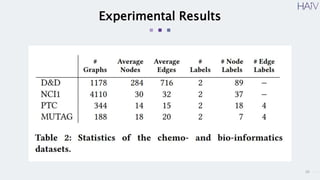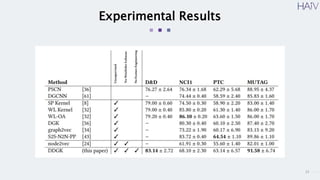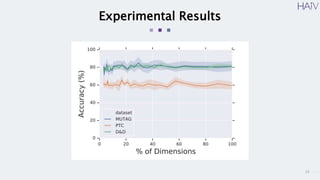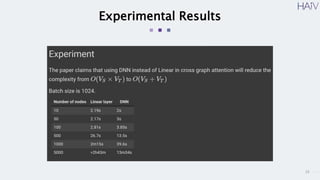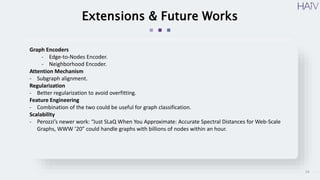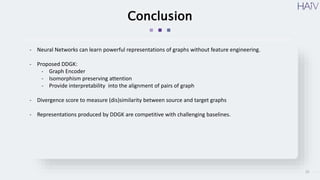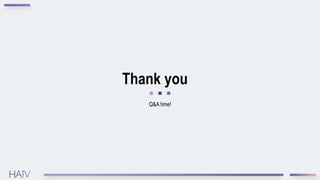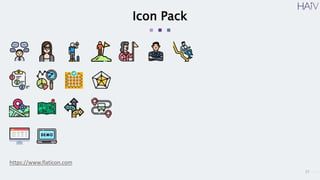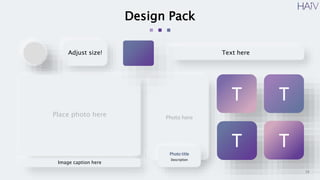1 sur 28
Publicité

### DDGK: Learning Graph Representations for Deep Divergence Graph Kernels

1. 2023.03.21 DDGK: Learning Graph Representations for Deep Divergence Graph Kernels Rami Al-Rfou, Dustin Zelle, and Bryan Perozzi WWW ‘19 Nguyen Minh Duc
2. Contents • Introduction • Related Works • Model Description • DDGK Algorithm • Experimental Results • Extensions and Future Works • Conclusion
3. 3 Introduction - Graph representation learning usually relies on - Supervised learning - Feature engineering - Generic representations of graphs - Algorithmic approach - Graph similarity measure is hard due to - NP-hard - Graph isomorphism - DDGK learns without supervision and domain knowledge
4. 4 Contributions Deep Divergence Graph Kernels (DDGK) Isomorphism Attention Experimental Results
5. 5 Related Works Traditional Graph Kernels: - Graph Edit Distance (Gao, et al., 2010) and Maximum Common Subgraph (Bunke, et al., 2002) - Weisfeiler-Lehman Graph Kernels (Kriege, et al., 2016) Node Embedding Methods: - DeepWalk (Perozzi, et al., 2014) - Graph Attention (Abu-El-Haija, et al., 2018) Graph Statistics (Feature engineering): - NetSmilie (Berlingerio, et al., 2012) - DeltaCon (Koutra, et al., 2013) Supervised Graph Similarity - CNN for graphs (Niepert, et al., 2016) - Graph Convolutional Networks (T. Kipf and M. Welling, 2016)
6. 6 Model Description Node-To-Edges Encoder Input: A one-hot encoded vertex Output: The vertex’s neighbor Consists of Fully connected DNN Modeled as a Multi-Label Classifier Graph encoding 1
7. 7 Model Description Isomorphism Attention Given two graphs 𝑆 (Source graph) and 𝑇 (Target graph) Provides a bidirectional mapping across the pair’s nodes Input: A one-hot encoded vertex from 𝑇 Output: The vertex’s neighbor Cross-Graph Attention 2
8. 8 Model Description Cross-Graph Attention 2 The first attention network (𝑀𝑇→𝑆 ) Place photo here Assigns every node in 𝑇 with a probability distribution over the nodes of 𝑆 Consists of one Linear layer Modeled as a multiclass classifier 𝑃𝑟 𝑣𝑗 𝑢𝑖 = 𝑒𝑀𝑇→𝑆(𝑣𝑗,𝑢𝑖) 𝑣𝑘∈𝑉𝑆 𝑒𝑀𝑇→𝑆(𝑣𝑘,𝑢𝑖)
9. 9 Model Description Cross-Graph Attention 2 The reverse attention network (𝑀𝑆→𝑇 ) Place photo here Maps the neighborhood in 𝑆 to the neighborhood in 𝑇 Consists of one Linear layer Modeled as a multilabel classifier 𝑃𝑟 𝑢𝑗 𝑁(𝑣𝑖) = 1 1 + 𝑒−𝑀𝑆→𝑇(𝑢𝑗,𝑁 𝑣𝑖 )
10. 10 Model Description Cross-Graph Attention 2 Isomorphism Attention Place photo here
11. 11 Model Description Node attribute regularizer Attributes Consistency 3 Attribute distribution over nodes Vertices and edges could have their own attributes Cross-Graph attention could provide several equally good mapping Solution: adding regularizing losses to preserve nodes and edges attributes Replace 𝑄𝑛 with 𝑄𝑒, we obtain Edge Attribute Regularizer
12. 12 DDGK Algorithm Parameter specification The Algorithm 1
13. 13 DDGK Algorithm Train source graph encodings The Algorithm 1
14. 14 DDGK Algorithm Train the Cross-Graph Attention The Algorithm 1
15. 15 DDGK Algorithm Save the similarity score in the matrix 𝚿 for every pair of source and target graph The Algorithm 1 Could be used as a representation vector
16. 16 DDGK Algorithm - Since Ψ is not a perfect function, 𝐷(𝑆| 𝑆 ≠ 0 could happen. - Setting 𝐷(𝑆| 𝑇 ≔ 𝐷(𝑆| 𝑇 − 𝐷(𝑆||𝑆) ensures 𝐷(𝑆| 𝑆 = 0 - If symmetry is required, we can define 𝐷(𝑆| 𝑇 ≔ 𝐷(𝑆| 𝑇 + 𝐷(𝑇||𝑆) Graph Divergence 2
17. 17 DDGK Algorithm DDGK requires 𝑂(𝑇𝑁2 𝑉) computations, where 𝑇 = max(𝜌, 𝜏) 𝑁 = The number of graphs 𝑉 = The average number of nodes Linear layers in Cross-Graph Attention could be replaced by a DNN with fixed size hidden layers to reduce the network size from 𝑂( 𝑉𝑆 × 𝑉𝑇 ) to 𝑂( 𝑉𝑆 + 𝑉𝑇 ) Scalability 3 For large number of source graphs, we could sample 20% of them and DDGK could still achieve high accuracy
18. 18 Experimental Results
19. 19 Experimental Results
20. 20 Experimental Results
21. 21 Experimental Results
22. 22 Experimental Results
23. 23 Experimental Results
24. 24 Extensions & Future Works Graph Encoders - Edge-to-Nodes Encoder. - Neighborhood Encoder. Attention Mechanism - Subgraph alignment. Regularization - Better regularization to avoid overfitting. Feature Engineering - Combination of the two could be useful for graph classification. Scalability - Perozzi’s newer work: “Just SLaQ When You Approximate: Accurate Spectral Distances for Web-Scale Graphs, WWW ’20” could handle graphs with billions of nodes within an hour.
25. 25 Conclusion - Neural Networks can learn powerful representations of graphs without feature engineering. - Proposed DDGK: - Graph Encoder - Isomorphism preserving attention - Provide interpretability into the alignment of pairs of graph - Divergence score to measure (dis)similarity between source and target graphs - Representations produced by DDGK are competitive with challenging baselines.
26. Thank you Q&A time!
27. 27 Icon Pack https://www.flaticon.com
28. 28 Design Pack Adjust size! Image caption here Place photo here Text here Photo here Photo title Description T T T T

### Notes de l'éditeur

1. Generic representations of graphs -> Generic node alignment -> Extract useful information Algorithmic approach from theoretical computer science NP-hard natural of the classical measurement such as Graph Edit Distance, and Maximum Common Subgraph Graph isomorphism is a hard problem (no polynomial algorithm)
2. DeepWalk learns embeddings of a graph's vertices, by modeling a stream of short random walks
3. Overfit the model on the source graph to accurately obtain the graph’s structure
4. Similar idea with Target Graph
5. The idea is given a vertex in the target graph, find the most similar vertex from the source graph Activation layer is Softmax
6. The source graph encoder outputs the neighbors of the chosen vertex From that, the reverse attention predict its corresponding position in the target graph Activation layer: Sigmoid
7. Overall structure of the model
8. There could be a lot of node mappings from the target to the source graphs. But not all of them preserve the attributes on the graph’s nodes and edges. Solution?
9. This is to demonstrate the power of attribute regularization. They are two identical graph, and the attention map should produce an Identity matrix
10. This is one application of DDGK, Hierarchical Clustering. 30 different graphs Graphs are sampled from different data sets such as neural network structure, social network, network of common nouns and adjectives in a novel, and chemistry-related graph.
11. Dimension sampling. Experiment with different amount of sampling in the source graph set. You can notice that the accuracy converges quickly from just 20% of the original size.
12. I also did my own experiment on this method I implemented this model on Google Colab and measure the time taken to process graphs of different sizes.
13. SLaQ uses spectral analysis on graph, which relies on some linear algebra properties of graph. I have looked at this paper but it’s quite hard to understand.
Publicité